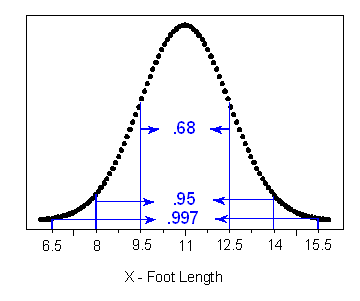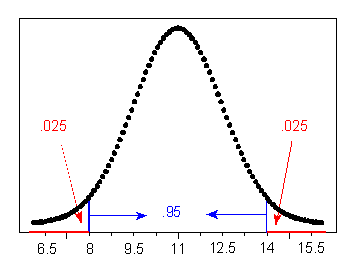## Normal Random Variables (3 of 6)

### Learning Objectives

• Use a normal probability distribution to estimate probabilities and identify unusual events.

## The Empirical Rule in a Context

Suppose that foot length of a randomly chosen adult male is a normal random variable with mean $\mathrm{μ}=11$ and standard deviation $\mathrm{σ}=1.5$ . Then the empirical rule lets us sketch the probability distribution of X as follows:• (a) What is the probability that a randomly chosen adult male will have a foot length between 8 and 14 inches?
• (b) An adult male is almost guaranteed (0.997 probability) to have a foot length between what two values?
• Answer: 6.5 and 15.5 inches
• (c) The probability is only 2.5% that an adult male will have a foot length greater than how many inches?

Ninety-five percent of the area is within 2 standard deviations of the mean, so 2.5% of the area is in the tail above 2 standard deviations. The x-value 2 standard deviations above the mean is 14 inches.Now you should try a few: questions (d), (e), and (f) are presented in the Learn By Doing activity. Use the figure preceding question (a) to help you.

### Learn By Doing

Comment

Notice that there are two types of problems we may want to solve: those like (a) and, from the Learn By Doing activity, (d) and (e), in which a particular interval of values of a normal random variable is given and we are asked to find a probability; and those like (b), (c), and, from the Learn By Doing, (f), in which a probability is given and we are asked to identify values of the normal random variable.